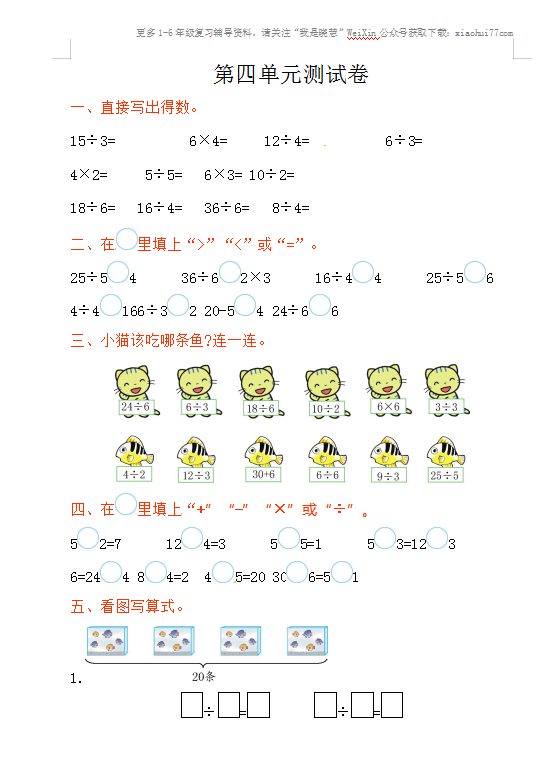15÷3=     6×4=         12÷4=　　6÷3=

4×2=     5÷5=           6×3=            10÷2=

18÷6=   16÷4=        36÷6=            8÷4=